# Recurrent Neural Network

## Recurrent Neural Network (RNN)

In this article, I am going to discuss Recurrent Neural Networks (RNNs). In the domains of AI, machine learning, and deep learning, neural networks simulate how the human brain functions, enabling computer systems to discover patterns and resolve challenges.

The neural network style known as Recurrent Neural Networks (RNNs) can be used to model sequence data. The behavior of RNNs, which are built from feedforward networks, is comparable to that of human brains.

Other algorithms are unable to predict sequential data in the same manner as recurrent neural networks do. Recurrent Neural Networks (RNNs) are the first machine learning algorithm to have an internal memory that retains information about its input, making it ideal for problems involving sequential data.

In conventional neural networks, all the inputs and outputs are independent of one another. However, in some situations, like when predicting the next word of a sentence, the prior words are important and must be remembered. The outcome was the development of RNN, which used a Hidden Layer to solve the issue. The Hidden state of an RNN, which retains precise details about a sequence, is its most crucial part.

All the data related to the calculations are stored in the memory of RNNs. It uses the same settings for each input because it generates the same result by carrying out the same operation on all inputs or hidden layers.

Depending on the issue you are trying to solve, many RNN architectures are possible. ranging from systems with a single input and output to ones with several (with variations between). Here are the types –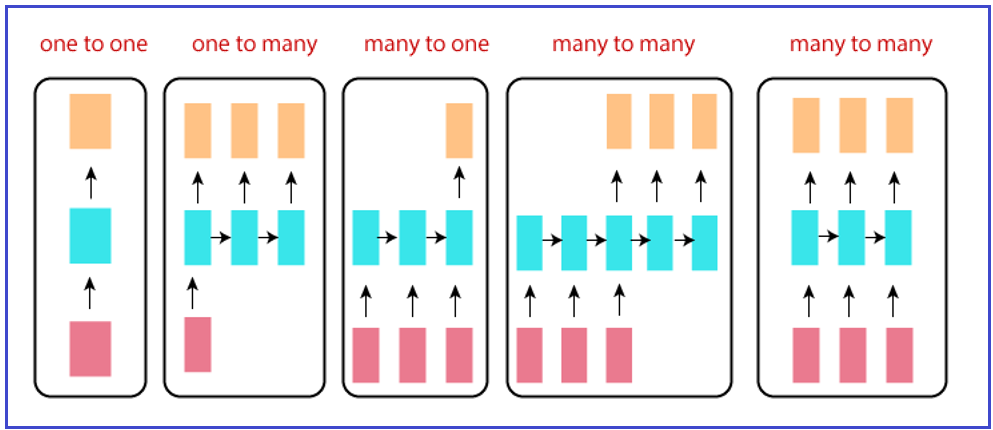1. One to One: Traditional neural networks employ a one-to-one architecture.
2. One to Many: In a one-to-many network, a single input could lead to a variety of outputs. The number of networks needed to create music is excessive.
3. Many to One: Combining numerous inputs from various time stages results in a single output. Such networks are used for sentiment analysis and emotion recognition, where the class label is determined by a word list.
4. Many To Many: There are a lot of choices for many to many. Three outputs result from two inputs. systems for automatic translation.
##### Applications of RNN (Recurrent Neural Network)-

In order to solve a range of problems involving sequence data, recurrent neural networks are frequently used. Sequence data can take many various forms, but the following are the most typical: Sequences of biological data, text, and video. You can work through a number of issues using RNN models and sequence datasets, including:

1. Speech recognition
2. Machine translation
3. Video action analysis
4. DNA sequence analysis
##### RNN (Recurrent Neural Network) Architecture and Working

RNNs have a “memory” that retains all data related to calculations. It executes the same action on all of the inputs or hidden layers to produce the output, using the same settings for each input.

An RNN is a kind of neural network that has hidden states and enables the use of previous outputs as inputs. Typically, they go like this: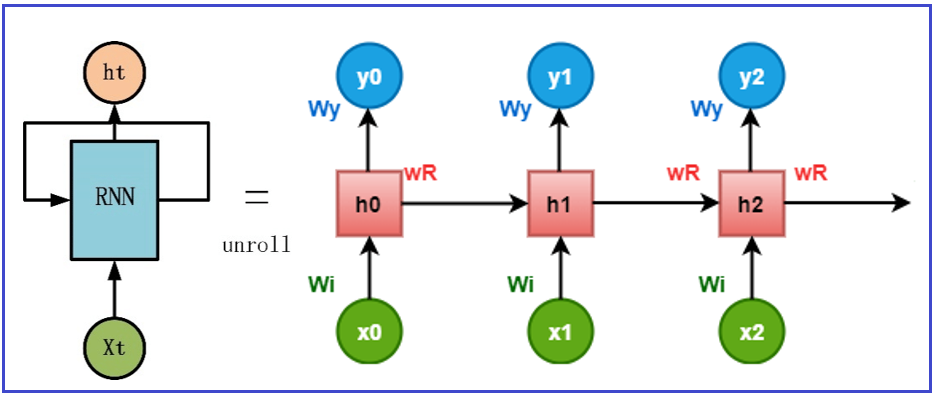As depicted in the first diagram of the previous picture, a straightforward RNN features a feedback loop. To create the second network in the above picture, the feedback loop seen in the 1st block can be unrolled this way. The following notation is used in the figure:

1. Xt is the input at timestamp t. We assume that it is a scalar value with a single feature.
2. Yt is the network’s output at the current timestamp t.
3. ht is the hidden states/units value at time stamp t. is the starting value of the vector.
4. Wt is recurrent layer weights connected to inputs.
5. Wr is weighted in the recurrent layer connected to hidden units.
6. Wy is weights connected to output units connected with hidden units.
7. b is the bias

We can unfold the network for time stamp t to obtain the output for time stamp t+1. The unfolded network and the feedforward neural network are quite similar. As an illustration, consider an activation function f: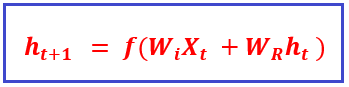The output at timestamp t will be –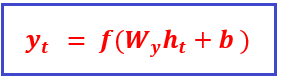As a result, in an RNN’s feedforward pass, the network calculates the hidden units’ values as well as the output after time stamps. The network’s related weights are shared throughout time. There are two sets of weights for each recurrent layer: one for the input and the other for the hidden unit.

##### Working of RNN (Recurrent Neural Network)

So, we can brief the working of RNN as –

Before sending the input to the middle layer of the neural network, the input layer x receives and analyses it.

The middle layer h contains several hidden layers, each with a unique set of activation functions, weights, and biases. If the individual hidden layer parameters are not affected by the hidden layer before it, or if the neural network has no memory, then you can use a recurrent neural network.

The Recurrent Neural Network will standardize the various activation functions, weights, and biases to guarantee that each hidden layer has the same properties. It will just create one hidden layer and loop over it as many times as necessary rather than building several.

##### Long Short-Term Memory (LSTM) Networks

An enhanced RNN, or sequential network, called a long short-term memory network, permits information to endure. Due to diminishing gradients, RNN has the flaw of being unable to recall long-term dependencies. Long-term dependency issues are specifically avoided when designing LSTMs.

They are currently frequently used and perform incredibly well when applied to a wide range of issues.

Intentionally, LSTMs are created to prevent the long-term reliance issue. They do not struggle to learn; rather, remembering information for extended periods of time is basically their default behavior. Like RNNs, LSTMs also feature a chain-like structure, but the repeating module is built differently. There are three neural network layers instead of just one, and they interact in a unique way.

These three LSTM layers are known as gates – The Forget gate, Input gate, and Output gate respectively. The first section determines whether the information from the preceding timestamp needs to be remembered or can be ignored. The cell attempts to learn new information from the input to this cell in the second section. The cell finally transmits the revised data from the current timestamp to the next timestamp in the third section.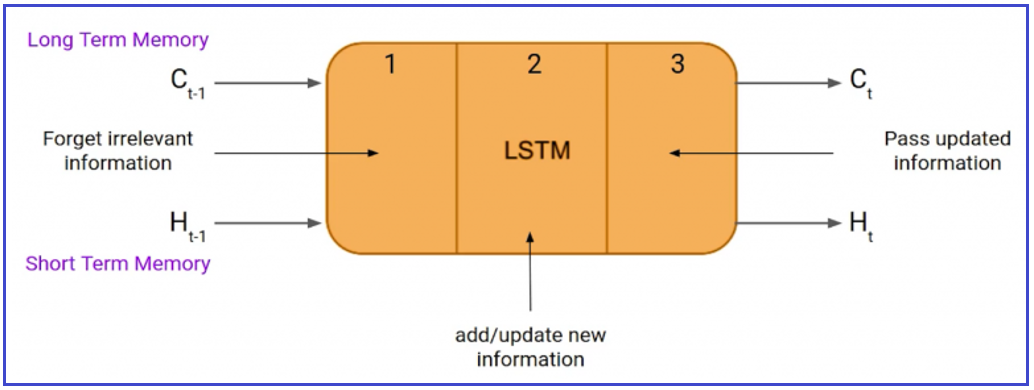An LSTM has a hidden state, just like a straightforward RNN, with H(t-1) standing for the hidden state of the prior timestamp and Ht for the hidden state of the present timestamp. Additionally, LSTMs have a cell state that is denoted by the timestamps C(t-1) and C(t), which stand for the prior and current timestamps, respectively.

In this case, the cell state is referred to as the long-term memory, and the hidden state is the short-term memory. Let us understand the working of each gate –

##### Forget Gate –

The initial step in an LSTM network cell is to choose whether to keep or discard the data from the preceding timestamp. The forget gate equation is given below –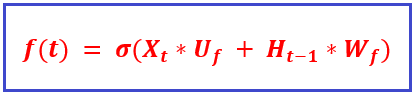Let us understand the equation –

1. Xt input at timestamp t
2. Uf weight corresponding to the input
3. Ht-1 hidden state for t-1
4. Wf weight matrix for hidden state

A sigmoid function is then put over it later. As a result, will become a number between 0 and 1. This is later multiplied by the cell state of the preceding timestamp.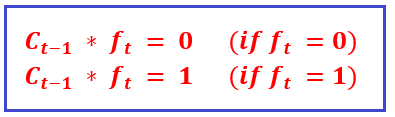The network will forget everything if is set to 0, but nothing if is set to 1.

##### Input Gate –

The value of the new information carried by the input is measured by the input gate. The input gate’s equation is shown below.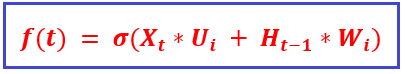Let us understand the equation –

1. Xt input at timestamp t
2. Uf weight corresponding to the input
3. Ht-1 hidden state for t-1
4. Wi weight matrix for hidden state

The equation of new information can be expressed as –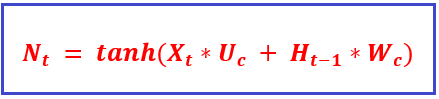The new data that had to be sent to the cell state now depends on a hidden state at timestamp t-1 in the past and input x at timestamp t. Tanh is the activation function in this case. The tanh function causes the value of fresh information to range from -1 to 1. The information is deducted from the cell state if the value is negative, and added to the cell state at the current timestamp if the value is positive. Now new information will not be added directly to the cell state. Thus, the updated equation can be expressed as –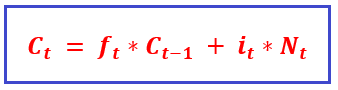In this case, Ct-1 represents the cell state at the current timestamp, and the other variables are those we previously calculated.

##### Output Gate –

The Output gate’s equation, which is quite similar to the equations for the two earlier gates, is shown below.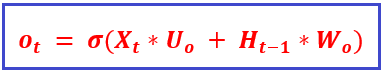Due to this sigmoid function, it will also have a value between 0 and 1. We will now use Ot and tanh of the updated cell state to determine the current hidden state. as displayed below.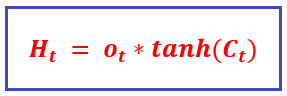It turns out that the hidden state depends on both the present output and long-term memory (Ct). Simply activate SoftMax on hidden state Ht if you need to take the output of the current timestamp.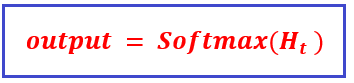##### Stock Price Prediction using RNN and LSTM

The future price prediction for various stocks using RNN and LSTM is demonstrated in this case study. Here, we’ll import data from various stocks using the Python Yfinance package. We can retrieve historical market data from the Yahoo Finance API using the Python library Yfinance. Using yfinance makes obtaining data for all Python developers really simple. Install the yfinance package first using –

pip install yfinance

```# Import necessary libraries
import numpy as np
import pandas as pd
import math
import sklearn
import sklearn.preprocessing
import datetime
import os
import matplotlib.pyplot as plt
import tensorflow as tf
from keras.models import Sequential
from keras.layers import Dense, LSTM, SimpleRNN
```
```#import yfinance
import yfinance as yf

#Collect data
```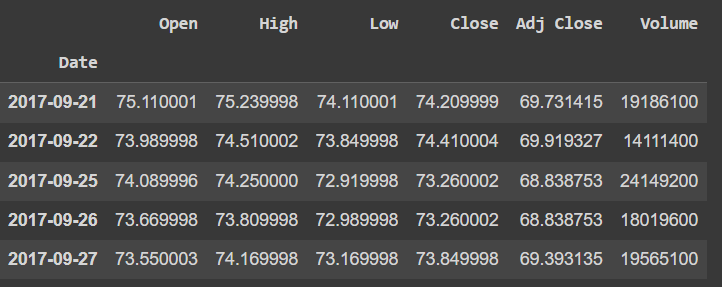###### # Check the details of dataset

stocks_data.describe()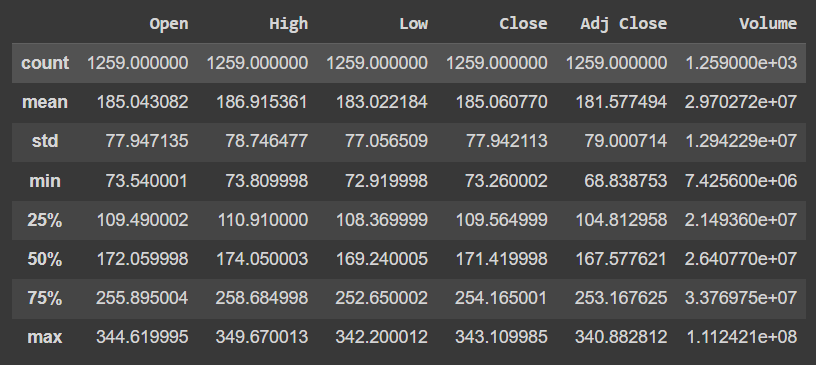Once the data has been gathered, we must choose the necessary column. The data includes historical information for the stock’s Open, Close, Low, High, Volume, and Adjusted Close. Adjusted close will be used to find patterns and make predictions. Additionally, divide the data into a train set and a test set so that we may subsequently assess our model.

Before moving on, we must construct some functions for downscaling data and turning data into a series of patterns that are followed for a specific price. We will utilize the MinMaxScaler tool in ScikitLearn to scale down.

```#Scaling Dataset
#Import scaler and initialise it
from sklearn.preprocessing import MinMaxScaler
scaler = MinMaxScaler(feature_range=(0,1))
#transform by converting it to array and shape of (-1,1)
data_scaled = scaler.fit_transform(np.array(target_data).reshape(-1,1))
#plot the scaled version of data
plot_scaled = pd.DataFrame(data_scaled).plot()
data_scaled
```

This is what the scaled data looks like –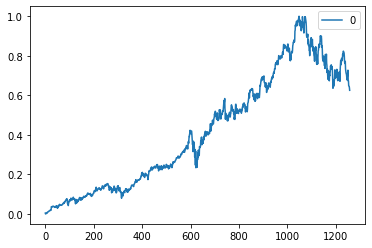We will now split our dataset into tests and train with an 80% training size.

```#Create the training data set
#Crete the scaled training set
train_data_len = math.ceil(data_scaled.shape*0.8)
train_data = data_scaled[0:train_data_len , :]

#Split the data into x_train and y_train data sets
x_train = []
y_train = []

for i in range(120, len(train_data)):
x_train.append(train_data[i-120:i, 0])
y_train.append(train_data[i,0])

#Create the testing data set
test_data = data_scaled[train_data_len - 120: , :]

#Create the data sets x_test and y_test
x_test = []
y_test = data_scaled[train_data_len:, :]
for i in range(120, len(test_data)):
x_test.append(test_data[i-120:i, 0])

x_train = np.array(x_train)
x_test = np.array(x_test)
y_train = np.array(y_train)
y_test = np.array(y_test)
```

Now, first, we will create an RNN model and evaluate the results generated by it.

```#Build the LSTM model
model = Sequential()

#compile the model

#Train the model
model.fit(x_train, y_train, batch_size=1, epochs=1)
```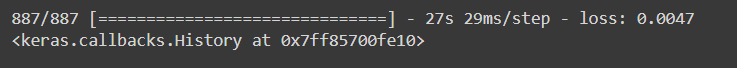```#Get the model predicted price values
predictions = model.predict(x_test)
predictions = scaler.inverse_transform(predictions)
#Get the root mean squared error (RSME)
rmse = np.sqrt( np.mean( predictions - y_test )**2 )
rmse
```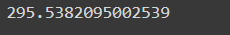```#plot the data
train = target_data[:train_data_len]
valid = target_data[train_data_len:]
valid['Predictions'] = predictions

#Visualize the data
plt.figure(figsize=(16,8))
plt.title('Model')
plt.xlabel('Date', fontsize=18)
plt.ylabel('Adj Close price USD (\$)', fontsize=18)
plt.legend(['Train', 'Val', 'Predictions'], loc='lower right')
plt.show()
```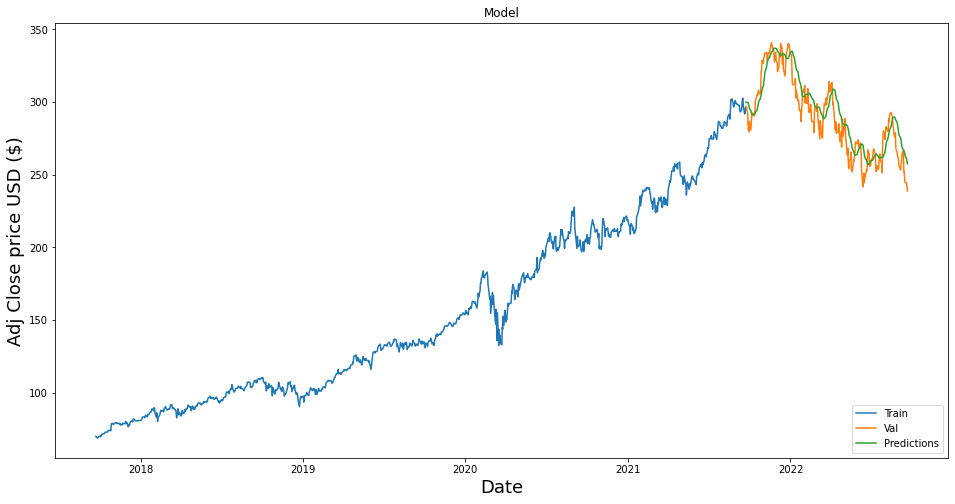Now, let us create an LSTM model and evaluate the results generated by it.

```#Build the LSTM model
model_LSTM = Sequential()

#compile the model

#Train the model
model_LSTM.fit(x_train, y_train, batch_size=1, epochs=1)
```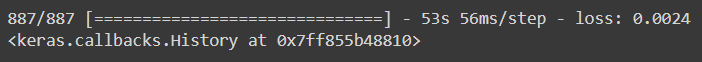```#Get the model predicted price values
predictions_LSTM = model_LSTM.predict(x_test)
predictions_LSTM = scaler.inverse_transform(predictions_LSTM)

#Get the root mean squared error (RSME)
rmse = np.sqrt( np.mean( predictions_LSTM - y_test )**2 )
rmse
```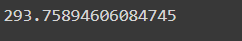```#plot the data
train = target_data[:train_data_len]
valid = target_data[train_data_len:]
valid['Predictions'] = predictions_LSTM

#Visualize the data
plt.figure(figsize=(16,8))
plt.title('Model')
plt.xlabel('Date', fontsize=18)
plt.ylabel('Close price USD (\$)', fontsize=18)